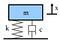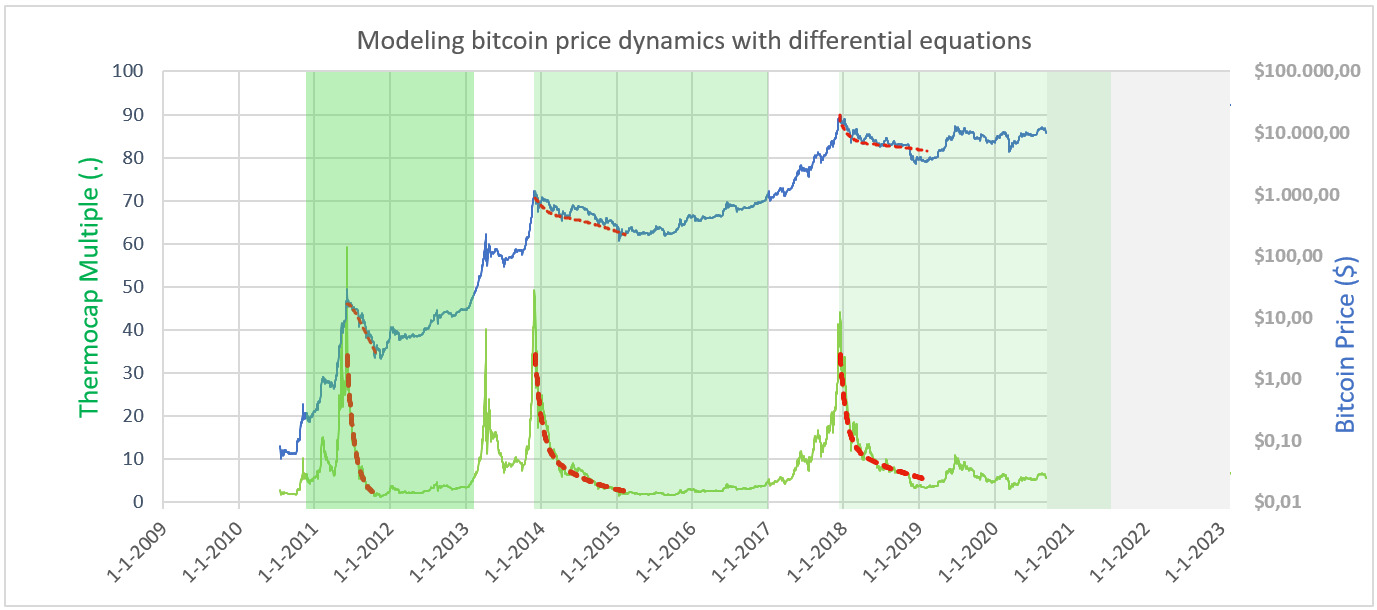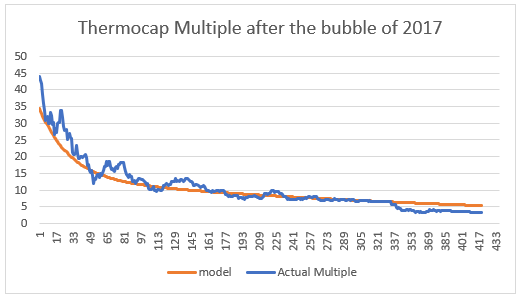# Modeling bitcoin price dynamics with systems dynamics theory

Introduction

During a so called ‘swine cycle’ of commodities, production increases when price increases. As investments in new production(mining) facilities take time to materialize, after a price crash, supply can stay on elevated levels for prolonged periods in time, depressing prices even more.

Bitcoins programmed supply enabling modelling as a dynamic system

Contrary to many other products or commodities, production of bitcoins is fixed and prescribed in the bitcoin protocol. Because of this, price dynamics doesn’t exhibit supply effects like a ‘swine cycle’. Therefore, bitcoin price dynamics might behave as a less complex human economic system in a bull run (or bubble/mania). Because of the simplicity, chances have increased to find reliable model parameters.

In this article, the method and its promising results are shown of modeling bitcoin price dynamics with system dynamics theory.

Building the signal to quantify price dynamics: The Sum of all miners production in US \$.

The “miners reward” is a summation of daily bitcoin reward denominated in US dollars.

1. First, the number of bitcoins mined is multiplied by the bitcoin price of that day. Example: 2010, 6th of august, the price was \$ 0.06. Number of bitcoins mined that day: 7350. So the miner reward was \$453. This is done for every day since bitcoin prices are known. It is not relevant whether these bitcoins were sold on the market.

2. Then, a summation is computed. The summation of all past bitcoin rewards of step 1 until that particular day is plotted as a data point. This is repeated for all days, resulting in the red line of above figure.

Another example: 6th of august, 2012, the reward was 11\$ x 7000 bitcoins = \$76 086. Cumulative rewards from the start of bitcoin production until that day: \$ 27.7 M\$.

Cumulative rewards up to ’20, sept 19th: 18 B\$. Currently(oct ’20), the daily reward is 900 bitcoin, price is 10 900, so every day, about 10 M \$ is added to the cumulative miners reward.

This Cumulative Bitcoin miner reward is plotted in red in above figure.

Dividing Bitcoin price and cumulative miner reward yields the so called Thermocap Multiple.

This multiple was first described by Nic Carter(ref. 1) and a continuously up-to-date graph can be found on www.glassnode.com.

Theoretical background for the Thermocap Multiple

It seems reasonable to consider this multiple as an underlying price mechanism. If the Market Cap is more than 50x the Miner cap, for évery miner, it makes a lot of sense to start selling. Therefore, it can be imagined that this is the reason for price pressure. In essence, the multiple differentiates compares investors and miners. A miner operates from a corporate structure: it usually has a balance sheet with debt, amortization and fixed costs. It’s P&L sheet is all about cash flow, profits and running costs. An investor however, mainly operates from a long term horizon, with fewer running costs(employees) and without debt. It actually owns the bitcoins. These investors include HODL-ers and since recently companies like MicroStrategy and Square.

The Thermocap Multiple compares miners business’ decisions with decisions made by investors.

Why use the Thermocap to investigate bitcoin price dynamics?

· It shows when a bubble in the price was present with a very high signal to noise ratio.

· On a linear scale, it clearly shows all periods of price bubbles. Contrary to the bitcoin price-time domain often used, there is no need for plotting the ThermoCap ratio on a logarithmic scale.

· It enables comparison of the bubble peaks.

· It appears to show a relatively constant value of the multiple for ‘healthy’ price levels.

· And finally: it appears to enable modeling of bitcoin price dynamics with second order dynamic systems.

Hypothesis

The cumulative behavior of all market participants in Bitcoin is a dynamic system. The ThermoCap multiple graph can be regarded as the response of this dynamic system. This response can be fitted with the generic solution of a step response from an overdamped dynamic system. What excites the dynamics of the bitcoin price is not the subject of this article.

Dynamic systems described with second order differential equations

Examples of such systems are a “resistor-inductor-capacitor circuit” and a “mass-spring-damper” system. They can be described with a 2nd order differential equation. Models like this are widely used in system dynamics & control and in mechanical & electrical engineering. 2nd order differential equations are occasionally used in economic papers as well.

Example(1): a dynamic system: an electrical circuit

The above electrical circuit can be described as:

where R = Resistor, L=Inductor, C=capacitor.

Example(2): A mass-spring-damper system.

This simple mass spring damper system is accurately described with the following differential equation:

where m=mass, c=damper, k=spring.A schematic of a mass, a spring and a damper. Only one direction(degree of freedom) is considered in this model

The mass influences the system with its inertia, the damper reacts with a counter-force when speed exceeds 0, and the spring produces a force when displacement exceeds 0.

The analytic solution of the response to an excitation

The response of the system to an excitation can be described as follows. Only the formulae of the overdamped response are given. More info can be found in (ref. 3) and (ref. 4)

Dynamic response for different damping ratios

Below responses are shown for different damping ratios. In this case, a ‘step’ in the displacement x. Imagine pulling the mass upward and letting it go. Depending on the damper coefficient, the mass and the spring constant, it takes longer or shorter for the mass to return to its equilibrium position. Moreover, these three values determine whether the mass oscillates, or not.

Fitting the right coefficients on bitcoin price dynamics

Based on the price dynamics shown in the Thermocap Multiple, the choice was made to model bitcoin price dynamics under the assumption of presence of an overdamped response in the Thermocap Multiple.

Three major peaks were selected to test model the model on accuracy to describe price dynamics. The three peaks are showing full retracement to low values of the Thermocap. These selected peaks are: June 2011, december 2013 and december 2017.

A response was fitted on the deflating of the bubble of 2011 and the values of the roots λ1, λ2 were found. As a first step, response on each individual bubble is fitted independently, resulting in the following graph.Fitting the three major bubbles assuming an overdamped response, with increasing damping coefficient.

In below graphs, the data ánd model of the multiple is plotted, using data from 2018 only.Thermocap multiple model, based on extrapolated roots from bubble of ’11 & ‘13

Fitting the roots from each bubble independently, suggests an assumption of a changing system. Only if the change can be traced back to a system variable, this can be acceptable. For example: the size of the Bitcoin market. The root λ1 decreases and thus the damping coefficient increases. The coefficients found in the model-fits approach the following values:

A varying root can be explained by the bitcoin market growth in both number of participants as professionality of the investors. So, as a second step, an attempt to describe the roots in bitcoin system variables was made. It turns out, that the root λ1 can be described using the bitcoin price(\$). The following rule was found:

The ζ values were also computed. It appears that indeed, the dynamic model exhibits increasingly overdamped behavior.

Interpretation of components in a bitcoin price dynamics

Consider the analogy with a mechanical mass-spring-damper-system. The larger the mass (e.g market cap), the higher the inertia. A “spring” like effect is present. If the price runs up violently, the price will crash violently. Furthermore, a “damping” or time dependency is clearly present. In a bear market, capitulation of the ‘weak hands’ happens gradually and according to a certain ratio of “weak hands” and “strong hands”. It is extremely interesting to see that apparently, the entire selling behavior follows a relatively predictable path.

Conclusion

• Bitcoin price dynamics was modeled with dynamic systems theory. Bitcoin price dynamics can be described with a 2nd order differential equation. The dynamics of the system can be described with an increasing damping coefficient that is directly related to the size of the Bitcoin market.
• The relevant ‘signal’ in Bitcoin price dynamics is the Thermocap Multiple.
• The model appears to describe a diminishing drop from peak to bottom.

Discussion

• It would be more rational to compare market capitalization with cumulative miner reward. However, fitting of the roots was found to become surprisingly constant, when accounting for increased supply.
• An explanation has been found for the increased length of bear markets after bubbles, because bitcoin dynamics is clearly dependent on the damping coefficient. As this coefficient increases with every higher price level, the length of the bear market increases with it.
• In case of a new bubble, with this model, an estimate can be made of the price level as function of time.

Critics

To be investigated

• Above model is not complete. It doesn’t consider the phase where excitation of the bitcoin price dynamics takes place. It might be caused by ‘supply shocks’: the halving of Bitcoin rewards every 4 years. This could be part of a next investigation.
• Modeling of bitcoin price dynamics assuming underdamped conditions. This approach might be feasible in the peaks in the thermocap before 2011 and during the peak in the middle of 2019.
• Investigating the same behavior in Gold. However, the “swine-cycle” might have to be accounted for.

References

1) https://www.docdroid.net/FbgH1WS/bitcoin-institution-riga-pdf#page=8 The first written document of the ThermoCap

2) https://studio.glassnode.com/metrics?a=BTC&m=mining.MarketcapThermocapRatio Real time Thermocap data.

3) https://www.researchgate.net/publication/227369071_Modeling_Price_Dynamics_in_eBay_Auctions_Using_Differential_Equations Research on the use of differential equations in economics.

4) https://rotorlab.tamu.edu/me617/handout2a_2008.pdf an accessible handout with explanation and exercises on the use of differential equations in mechanics

5) Franklin, Powell, Emami-Naeini, 2002, Feedback Control of Dynamic systems, ISBN-0–13–032393–4